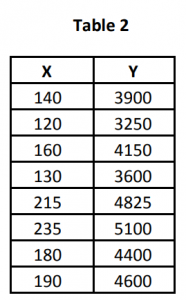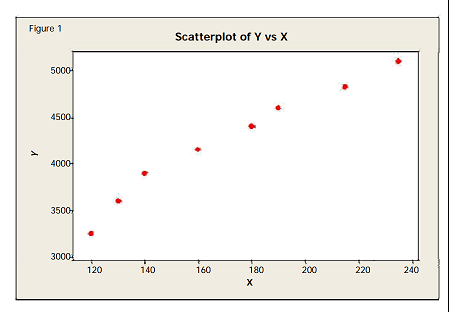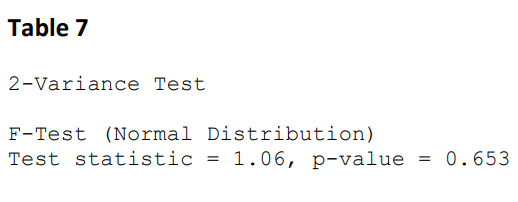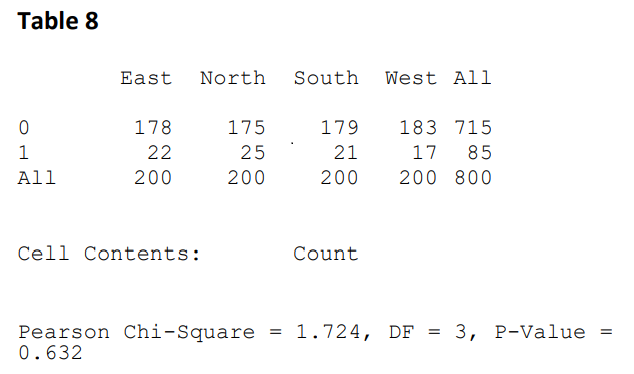India - +91 7838547711, US - +1 513 657 9333

## Lean Six Sigma Green Belt Sample Paper: Analyze

1. The Regression equation is Sales= 4430+4.63*Adspend. If Adspend \$ 4400, what is expected sales?
2. Type I error is
3. What is the Alternative Hypothesis in One‐Way ANOVA?
4. For the data provided in Table 2, please find Correlation coefficient of the two continuous variables and draw inferences5. Please comment on correlation between X and Y based on figure 16. If Confidence level=90%, which of the following statement is correct?
7. If continuous X and continuous Y are perfectly correlated, which of the following statements is most appropriate?
8. Six Sigma Green Belt wants to ascertain whether average transaction time post improvement has reduced. What is the most appropriate test that the GB should carry out? Consider the data to be independent and normally distributed?
9. What is the main deliverable of Analyze Phase?
10. What tool can help us determine the vital few out of trivial many?
11. If we want to compare two variances, what test should we carry out?
12. Based on the output in table 7, what is the most appropriate inference at a 5% significance level?13. The manager wants to compare average handling time of two teams. Which test should he use?
14. Refer to output in table 8, and choose the most appropriate response at 5% significance level15. Which hypothesis test is used to compare averages of more than two populations?
16. Which of the following is NOT one of the ‘M’s in 6 Ms?
17. In FMEA, which numerical measure represents - "how serious is the effect of the failure"?
18. Risk Priority Number is the product of
19. Which of the following is correct about Regression analysis?
20. What will be the Confidence Level and Power, if beta =15%, and alpha = 10%?
21. Process maps can be analyzed for: